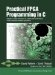# CO_BIT_INSERT

` int32 co_bit_insert(int32 destination,                     uint8 dest_start_bit,                     uint8 num_bits,                     int32 source); `

co.h

#### Description

This function provides a concise and efficient method of inserting bits into a 32-bit signed integer.

#### Arguments

The arguments for co_bit_insert are as follows:

 int32 destination The 32-bit signed integer into which the bits are inserted. uint8 dest_start_bit The bit position in the destination value where the bits will begin to be inserted, from dest_start_bit toward the most-significant bit. uint8 num_bits The number of bits to extract from the source, starting at the least-significant bit. int32 source The source of the bits to be inserted.

#### Return Value

Returns a 32-bit signed integer (int32) representing the original destination value, but with num_bits bits, starting at bit location dest_start_bit, replaced with the first num_bits bits of the source integer.

#### Notes

The co_bit_insert, co_bit_insert_u, co_bit_extract, and co_bit_extract_u functions operate on 32-bit data. If required, you can truncate the input and results to suit your needs. (Values greater than 32 bits are currently not supported.) For example, if you are operating on 16-bit numbers, you can use the functions as follows:

` co_int16 src = 0xffff; co_int16 dest; dest = co_bit_extract(src, 8, 16); // dest == 0x00ff dest = co_bit_insert(src, 8, 8, dest); // dest == 0xffff `

In this example, src and dest automatically get promoted (and sign-extended, since they are signed values) to 32-bit integers when used as arguments. The return value gets cast invisibly to a co_int16, which just truncates 32 to 16 bits.Practical FPGA Programming in C
ISBN: 0131543180
EAN: 2147483647
Year: 2005
Pages: 208